Bonky Zhu
If someone is able to show me that what I think or do is not right, I will happily change, for I seek the truth, by which no one was ever truly harmed. It is the person who continues in his self-deception and ignorance who is harmed.

编译原理 – 词法分析

词法分析程序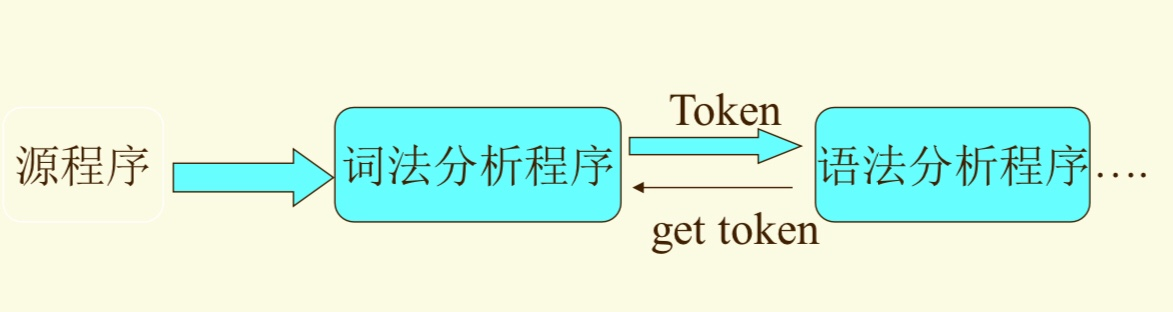词法分析程序的任务

• 读源程序，产生用二元组表示的单词符号
(单词种类，单词自身的值)。
单词种类是语法 分析需要的信息，单词自身的值是语义分析和代码 生成阶段需要的信息。
对某些单词来说，不仅仅需要它的值， 还需要其它信息以方便代码生成。
如标识符还需要记载它的层次，类别(整形、实形、布尔等)，这些属性都收集到一个符号表中。二元组无法描述这多信息，一般将词法分析输出的单词二元表示设计成(标识符,指向该标示符所在符号表中位置指针)。
• 滤掉空格，跳过注释、换行符
• 记录源程序的行号，以便出错处理程序准确
定位源程序的错误
• 宏展开等......

Lex可以根据一个你的词法规则，生成一个C语言写的的词法分析器。YACC可以生成一个语法分析器。

单词描述工具

⽤正规⽂法或者正则表达式描述单词符号的词法构成

正规表达式

1. $\epsilon$和中都是字母表上的正规式，对应正规集为${\epsilon}$和$\varnothing$；
2. 任何$a\in \sum$, $a$是$\sum$上的一个正规式，表示的正规集分别为${a}$；
3. 假设$e_1$和$e_2$，都是$\sum$上的正规式，表示的正规集分别为$L(e_1)$ 和$L(e_2)$，则$(e_1)$, $e_1|e_2$, $e_1\cdot e_2$, $e_1^*$,"都是$\sum$上的正规式，它们表示的正规集分别为$L(e_1)$，$L(e_1)\cup L(e_2)$ , $L(e_1)L(e_2)$, $(L(e_2)$

正规表达式于正则文法的转换

正则表达式转换为正规文法

• 选定⼀个⾮终结符S定为识别符号，⽣成规则 S→r；
• 对于xy是正则式，定义⼀个规则A→xy,然后改写成：A→xB, B→y
• 对于xy正则式，定义⼀个规则A→xy,然后改写成：A→xB|y, B→xB|y
• 对于x|y正则式，定义⼀个规则A→x|y

S→aA A→(a|d)* A→(a|d)B|$\epsilon$
B→(a|d)B|$\epsilon$ A→aB|dB|$\epsilon$ B→aB|dB|$\epsilon$

正规文法转换为正则表达式

• S=r；
• 对于A→xB, B→y规则，定义⼀个正则式A=xy,
• 对于A→xA|y规则，定义A=x*y正则式
• 对于规则A→x|y,定义A=x|y正则式

有穷自动机

DFA

M = ( S，Σ ，δ，$s_0$，F )

• S：有穷状态集。
• Σ：输入字母表，即输入符号集合。假设ε不是Σ中的元素。
• δ：将S×Σ映射到S的转换函数。 δ(s,a)表示 从状态s出发，沿着标记为a的边所能到达的状态
• $s_0$：开始状态 (或初始状态)。
• F：接收状态（或终止状态）集合。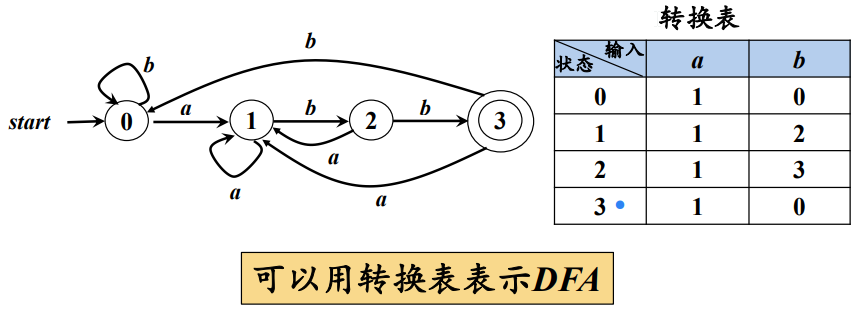理解：根据输入状态，下一个状态是固定的

NFA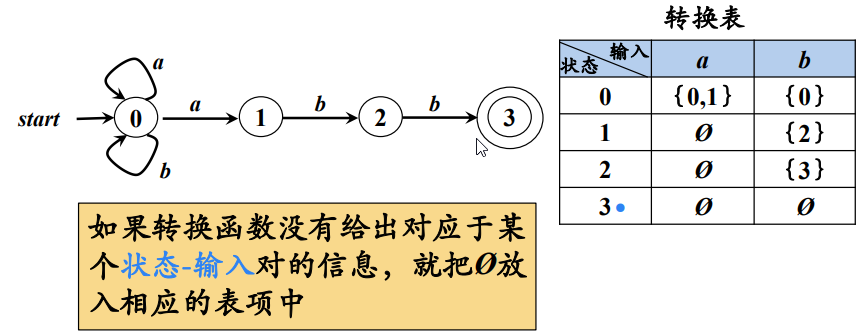带有ε的NFA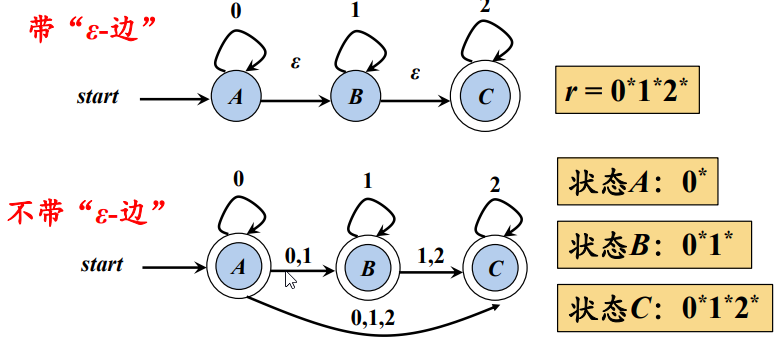正则表达式到NFA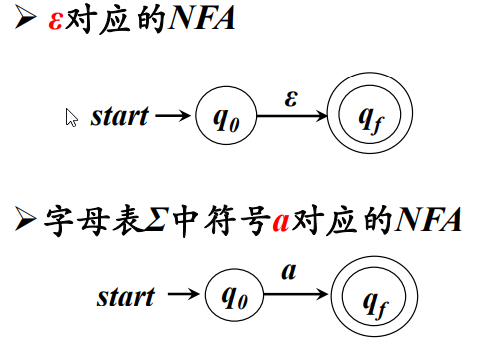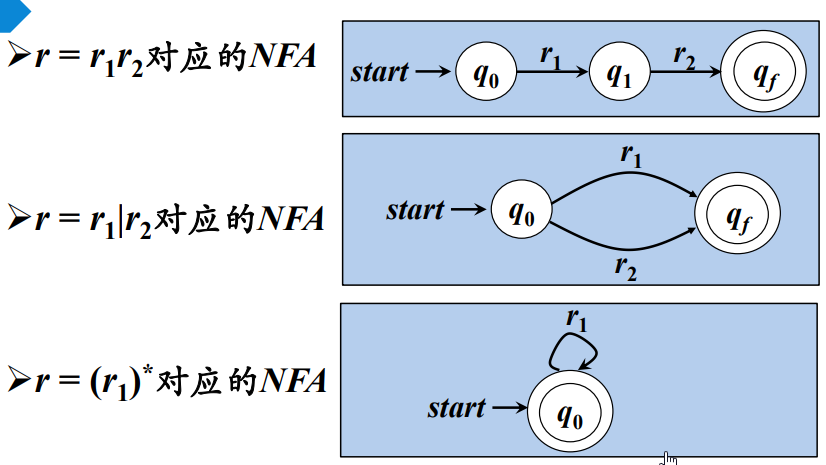NFA到DFA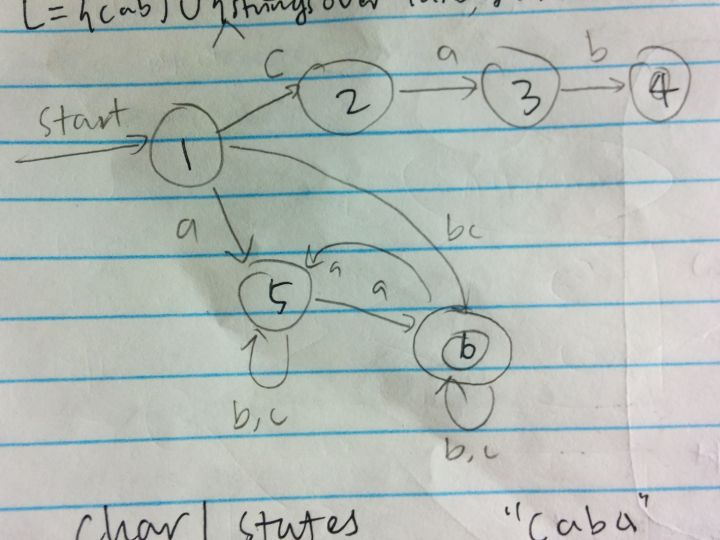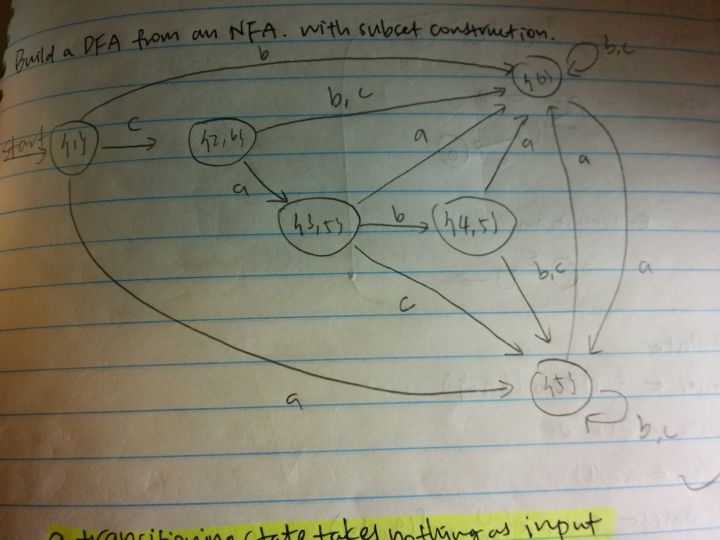DFA的化简

• I0 = 非状态元素构成的集合，I1 = 终态元素构成的集合
• 经过多次划分后，要保证，任意一个Ik中的元素通过move(Ik,某个字符)的结果都同属于一个Iz，这时候划分完成。否则把状态不同的单独划分出去。
• 重复上一步，直至没有新的I子集增加。
• 从子集中任选一个代替整体，画出最简DFA。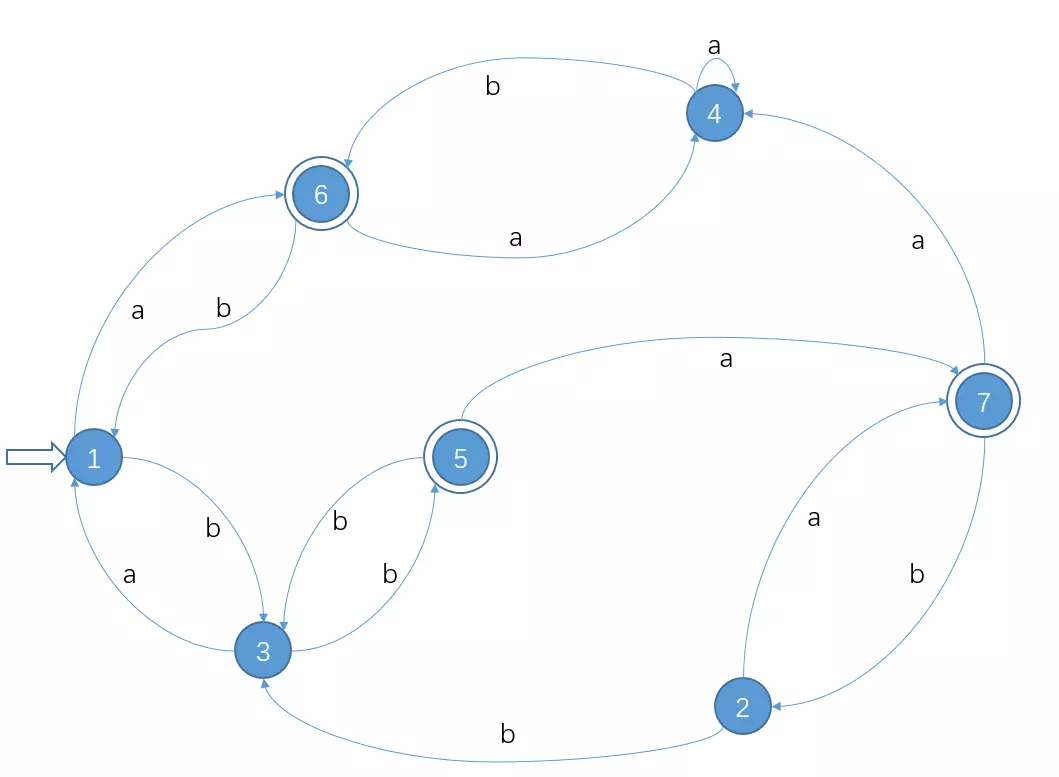-----------------I0、I1分割------------------- I0 = {1,2,3,4} ; 非终态 I1 = {5,6,7} ; 终态

move(1,a) = 6 ∈I1 move(1,b) = 3 ∈I0  move(2,a) = 7 ∈I1 move(2,b) = 3 ∈I0  move(3,a) = 1 ∈I0 move(3,b) = 5 ∈I1  move(4,a) = 4 ∈I0 move(4,b) = 6 ∈I1 可以发现，{1,2}是等价的,{3,4}是等价的 所以现在分割成了：I2 = {1,2}, I1 = {5,6,7}, I3 = {3,4}

move(1,a) = 6 ∈I1 move(1,b) = 3 ∈I3  move(2,a) = 7 ∈I1 move(2,b) = 3 ∈I3 可以发现，是等价的，不用分割

move(3,a) = 1 ∈I2 move(3,b) = 5 ∈I1  move(4,a) = 4 ∈I3 move(4,b) = 6 ∈I1 可以发现，不是等价的，分割成{3},{4} 所以现在分割成了：I2 = {1,2}, I1 = {5,6,7}, I4 = {3}, I5 = {4}

move(5,a)  = 7 ∈ I1 move(5,b)  = 3 ∈ I4  move(6,a)  = 4 ∈ I5 move(6,b)  = 1 ∈ I2  move(7,a)  = 4 ∈ I5 move(7,b)  = 2 ∈ I2  可以发现，不是等价的，分割成{6,7}, {5} 所以现在分割成了：I2 = {1,2}, I4 = {3}, I5 = {4}, I6 = {5},I7 = {6,7}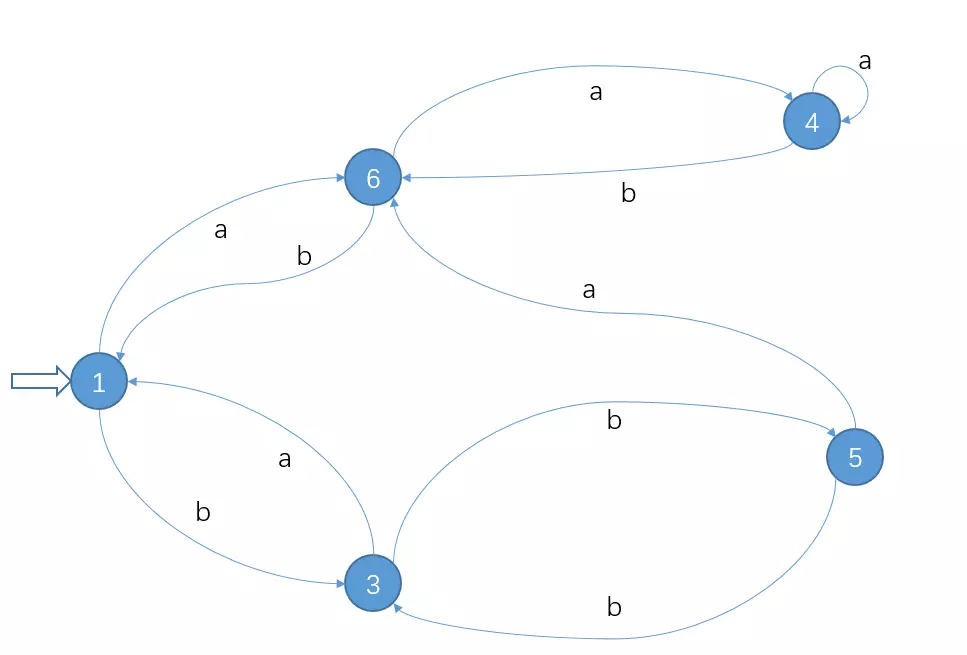Share